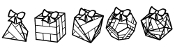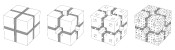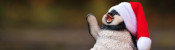mscroggs.co.uk
mscroggs.co.uksubscribe

# Blog

## Christmas card 2016

2016-12-20
Last week, I posted about the Christmas card I designed on the Chalkdust blog.
The card looks boring at first glance, but contains 12 puzzles. Converting the answers to base 3, writing them in the boxes on the front, then colouring the 1s green and 2s red will reveal a Christmassy picture.
If you want to try the card yourself, you can download this pdf. Alternatively, you can find the puzzles below and type the answers in the boxes. The answers will be automatically converted to base 3 and coloured...
 # Answer (base 10) Answer (base 3) 1 0 0 0 0 0 0 0 0 0 2 0 0 0 0 0 0 0 0 0 3 0 0 0 0 0 0 0 0 0 4 0 0 0 0 0 0 0 0 0 5 0 0 0 0 0 0 0 0 0 6 0 0 0 0 0 0 0 0 0 7 0 0 0 0 0 0 0 0 0 8 0 0 0 0 0 0 0 0 0 9 0 0 0 0 0 0 0 0 0 10 0 0 0 0 0 0 0 0 0 11 0 0 0 0 0 0 0 0 0 12 0 0 0 0 0 0 0 0 0
1. The square number larger than 1 whose square root is equal to the sum of its digits.
2. The smallest square number whose factors add up to a different square number.
3. The largest number that cannot be written in the form $$23n+17m$$, where $$n$$ and $$m$$ are positive integers (or 0).
4. Write down a three-digit number whose digits are decreasing. Write down the reverse of this number and find the difference. Add this difference to its reverse. What is the result?
5. The number of numbers between 0 and 10,000,000 that do not contain the digits 0, 1, 2, 3, 4, 5 or 6.
6. The lowest common multiple of 57 and 249.
7. The sum of all the odd numbers between 0 and 66.
8. One less than four times the 40th triangle number.
9. The number of factors of the number $$2^{756}$$×$$3^{12}$$.
10. In a book with 13,204 pages, what do the page numbers of the middle two pages add up to?
11. The number of off-diagonal elements in a 27×27 matrix.
12. The largest number, $$k$$, such that $$27k/(27+k)$$ is an integer.

### Similar postsChristmas card 2017Christmas card 2019Christmas card 2018Christmas (2019) is over

Comments in green were written by me. Comments in blue were not written by me.
@Matthew: Thank you for the prompt response! It makes sense now and perhaps I should have read a little closer!
Dan Whitman
@Dan Whitman: Find the difference between the original number and the reverse of the original. Call this difference $$a$$. Next add $$a$$ to the reverse of $$a$$...
Matthew
In number 4 what are we to take the difference between? Do you mean the difference between the original number and its reverse? If so when you add the difference back to the reverse you simply get the original number, which is ambiguous. I am not sure what you are asking us to do here.
Dan Whitman